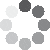Enter parameters Roof Calculator
Roof Width
ft
• inches (in)
• feet (ft)
• yards (yd)
• millimeters (mm)
• centimeters (cm)
• meters (m)
Angle
°
Rafter Thickness
in
• inches (in)
• feet (ft)
• yards (yd)
• millimeters (mm)
• centimeters (cm)
• meters (m)
Overhang
in
• inches (in)
• feet (ft)
• yards (yd)
• millimeters (mm)
• centimeters (cm)
• meters (m)
Ridge Thickness
in
• inches (in)
• feet (ft)
• yards (yd)
• millimeters (mm)
• centimeters (cm)
• meters (m)
Purlin Thickness
in
• inches (in)
• feet (ft)
• yards (yd)
• millimeters (mm)
• centimeters (cm)
• meters (m)
Gussets Base
in
• inches (in)
• feet (ft)
• yards (yd)
• millimeters (mm)
• centimeters (cm)
• meters (m)
Calculate Reset

Show sizes on the drawing
yes
• no
• yes
Units on the drawing
in
• inches (in)
• feet (ft)
• yards (yd)
• millimeters (mm)
• centimeters (cm)
• meters (m)
Colored drawing
colored
• black and white
• colored
Results
Truss Height:
0
in
• inches (in)
• feet (ft)
• yards (yd)
• millimeters (mm)
• centimeters (cm)
• meters (m)
Truss Width:
0
in
• inches (in)
• feet (ft)
• yards (yd)
• millimeters (mm)
• centimeters (cm)
• meters (m)
Real-time graphics. Make the calculations and see the changes.(3 votes, average: 5.00 out of 5)Loading...
Embed
Embed this calculator on your site!Add live graphics
Copied to clipboard! PreviewWhat's wrong?
Minimum 5 characters!